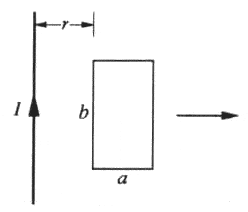# EMF about a loop moving toward a wire with current

## Homework Statement

A picture of a related problem is attached below. My problem is the same, except that v is toward the wire, not away from it. I, r, v, b and a are given."Find the emf about the loop without assuming that B changes at any point"

My problem is that this question isn't clear to me. Changes at any point of time, or point in space? And if a point in space, do they mean a point on my xy axis, or point inside the loop? The book gives the answer as
bvμI/(2π) * [1/r - 1/(r+a)], which I'm trying to figure out how to get.

## Homework Equations

emf = - d/dt ∫Bda

## The Attempt at a Solution

Here's how I would do the problem normally.
emf = - d/dt ∫ [μI / (2πx)] * [bdx], with limits of integration from r - vt to r - vt + a
= -bμI / (2π) * d/dt [ln(r-vt+a) - ln(r-vt)]
= -bvμI / (2π) * [1/(r - vt) - 1/(r - vt + a)]

This is the same as what the book got, but with t = 0, and the wrong sign . Can someone explain if this is correct, why t = 0, and how it follows from the question? I still don't get the "B doesn't change" condition".

Thanks.